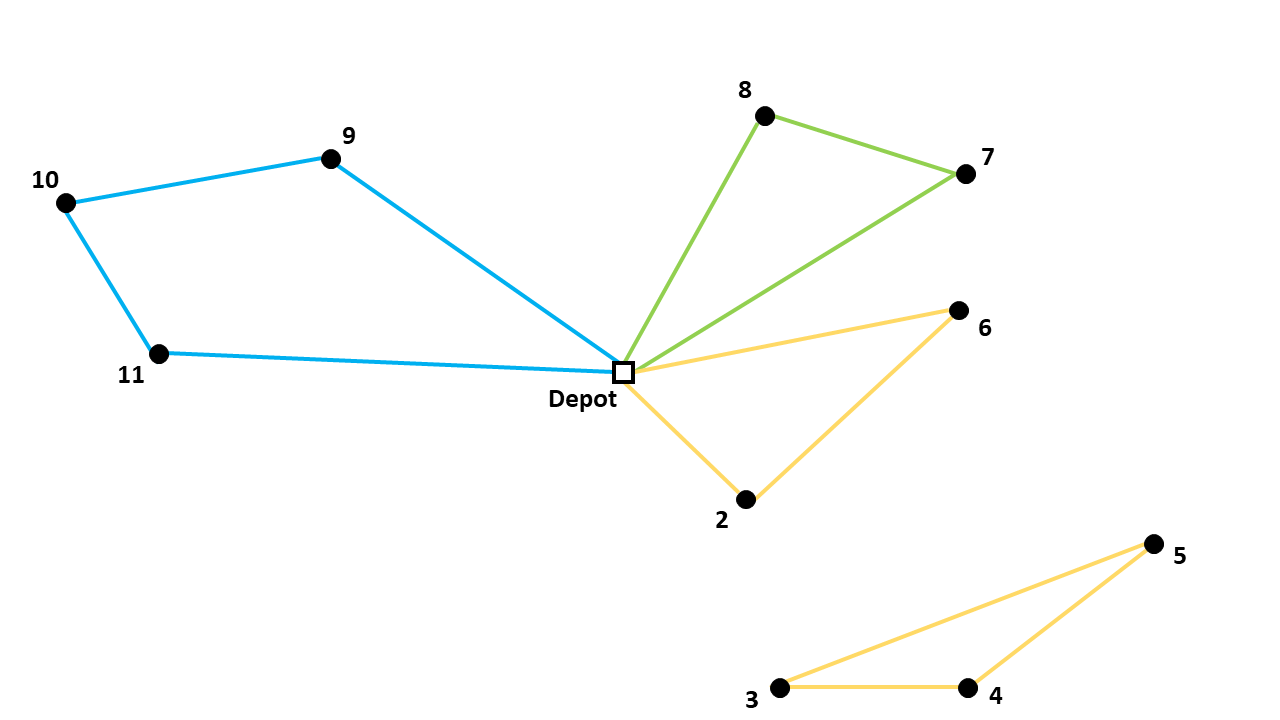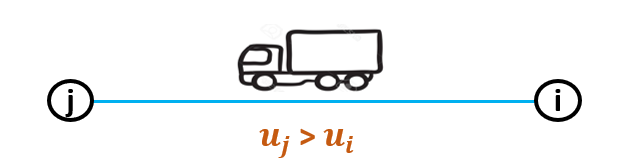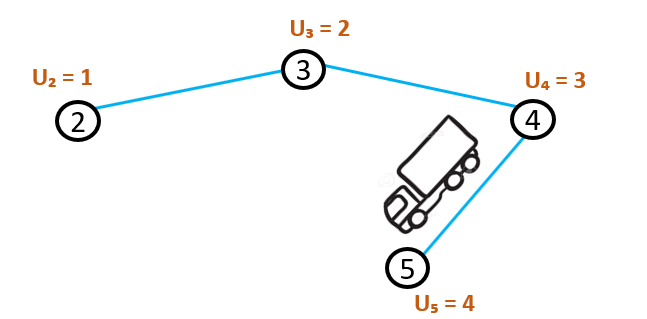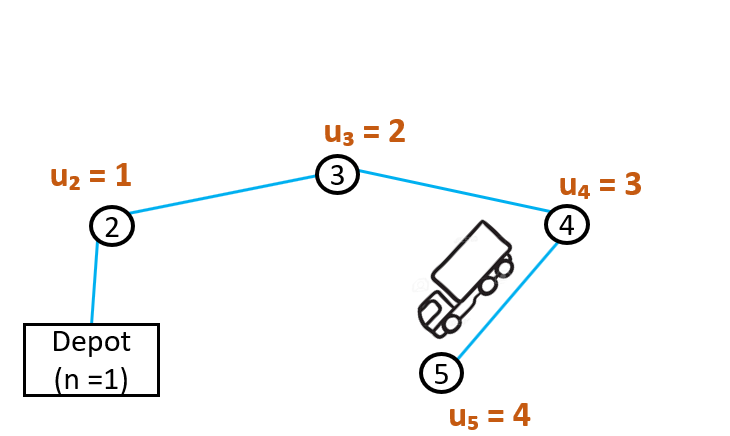# Miller-Tucker-Zemlin formulation

The Capacitated Vehicle Routing Problem Library contains four different formulation options. Those formulations use different methods for eliminating subtours. In this article, the Miller-Tucker-Zemlin formulation is discussed. Herein below an example of a subtour within a route for a CVRP:## Idea behind the formulation

The Miller-Tucker-Zemlin (MTZ) formulation uses an extra variable. The variable is called $$u_{i}$$ and gets a value for each node, except for the depot. If a vehicle drives from node i to node j, the value of $$u_{j}$$ has to be bigger than the value of $$u_{i}$$.So each time a new node is being visited, the value for $$u_{i}$$ increases.The node that the vehicle will visit after node 5, should again have a larger value of $$u_{i}$$. It would not be possible to go from node 5 to node 2, because that node already has a lower value of $$u_{i}$$. This ensures that a vehicle will not drive in a circle. Since that would make it impossible for every value of $$u_{i}$$ to be larger than the previous one. Since the depot does not get a value of $$u_{i}$$, it is possible to drive in a circle if the vehicle starts and ends at the depot.The vehicle can now drive from node 5 back to the depot and $$u_{j}$$ is always larger than $$u_{i}$$. So the only circles permitted to be driven are the ones passing the depot. All the other circles would be subtours and are eliminated by this formulation.

## Subtour Elimination Constraints

The binary variable $$x_{ijk}$$ has a value of 1 if vehicle k drives from node i to node j. $$Q$$ is the capacity of the vehicles and $$q_{i}$$ is the demand of node i. $$V$$ is a set containing all the nodes, and the depot is n=1. The constraints can be formulated as follows:

$u_{j} - u_{i} \geq q_{j} - Q (1 - x_{ijk}) \qquad \forall i,j \in V \setminus \{1\} \enspace i \neq j \qquad (1)$
$\qquad \qquad q_{i} \leq u_{i} \leq Q \qquad \forall i \in V \setminus \{1\} \qquad \qquad \qquad (2)$

If vehicle k drives from node i to node j, $$x_{ijk}$$ = 0 and constraint (1) can be rewritten to $$u_{j} \geq u_{i} + q_{j}$$. This ensure that the value of $$u_{j}$$ is at least $$q_j$$ more than $$u_i$$. So the value of $$u_j$$ is greater than the value of $$u_i$$

If vehicle k does not drive from node i to node j, the constraint is still valid. Constraint (1) could then be rewritten to $$u_{j} - q_{j} \geq u_i - Q$$. Constraint (2) states that $$q_j$$ is the lowest possible value of $$u_j$$ and $$Q$$ is the greatest possible value of $$u_i$$. So $$u_j-q_j$$ will at least be 0 and $$u_i-Q$$ will at most be 0. So $$u_j-q_j$$ is greater than or equal to $$u_i-Q$$

In the Capacitated Vehicle Routing Problem Library, the constraints are implemented in the section Miller Tucker Zemlin Section.